# Percolation analysis

This entry focuses on the application of percolation analysis to problems in statistical mechanics. For a general discussion see Refs.  

## Sites, bonds, and clusters

This topic concerns the analysis of connectivity of elements (sites) distributed in different positions of a given large system. Using some connectivity rules it is possible to define bonds between pairs of sites. These bonds can be used to build up clusters of sites. Two sites in a cluster can be connected directly by a bond between them or indirectly by one or more sequences of bonds between pairs of sites. The sites of the system can belong to different types (species in the chemistry language). Bonds are usually permitted only between near sites.

## Lattice and continuum (off-lattice) models

Attending to the spatial distribution of the sites, one can classify the models into lattice models and continuum (or off-lattice) models. Off-lattice models are more difficult to deal with from the numerical point of view, but in many applications they are expected to be more realistic than lattice models to capture the physics of a number of real systems  

## Connectivity rules

The connectivity rules (bonding criteria) that permit the creation of bonds can be of different nature: distance between sites, energetic interaction, types of sites, etc. In addition the bonding criteria can be either deterministic or probabilistic. In statistical mechanics applications one can find different bonding criteria, for example:

• Geometric distance: Two sites,$i$,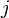$j$, are bonded if the distance between then satisfies: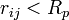$r_{ij} < R_p$. This criterium is used in the so-called site percolation models (see below).
• Probabilistic criteria: Two sites located at a certain distance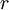$r$ are bonded with a given probability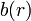$b(r)$, with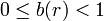$0 \le b(r) < 1$. This is the case of the so-called bond-percolation models.
• Energetic criteria (probabilistic): As an example, in the simulation of Ising models using cluster algorithms; two sites$i$,$j$, have a bonding probability given by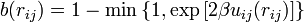$b(r_{ij}) = 1- \min \left\{ 1, \exp \left[ 2 \beta u_{ij}(r_{ij}) \right] \right\}$; where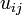$u_{ij}$ is the interaction energy between sites, and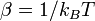$\beta = 1/ k_BT$ (See cluster algorithms for details).

## Percolation threshold

The sizes of the clusters of a given system depend on different control parameters: density and distribution of sites, bonding criteria (which could include the effect of temperature and energy interactions), etc.

Let us consider some initial conditions of the control parameters, in which, the bonding criteria leads to the formation of small clusters, i.e. all the cluster contain a small number of particles and the cluster size is much smaller than the linear dimension of the system. Now, if one varies gradually some control parameter(s) to increase the number of bonds in the system, then the number of clusters is expected to decrease, the number of sites per cluster and the cluster size will increase; and, eventually, the largest cluster size(s) (in one or several directions) will be similar to the overall system size (the system reaches the percolation threshold of the percolation transition).

### Percolation and boundary conditions

There are different possible criteria to consider that a cluster has percolated. The choice of percolation criteria usually depends on computational convenience, type of boundary conditions, dimensionality of the space, etc. In the particular case of considering periodic boundary conditions, a cluster realization is usually considered as percolating when, at least, one of the clusters becomes of infinite size (length) in, at least, one direction. This infinite size occurs, obviously, via the replication of the system that appears due to the periodic boundary conditions.

## Percolation and finite-size scaling analysis

### Example: Site-percolation on a square lattice

Let us consider a standard example of percolation theory,  a two-dimensional square lattice in which:

• Each site of the lattice can be occupied (by one particle) or empty.
• The probability of occupancy of each site is$\left. x \right.$, with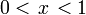$0 < \left. x \right. < 1$.
• Two sites are considered to be bonded if and only if:
• They are nearest neighbours and
• Both sites are occupied.

### Fraction of percolating realizations

On such a system, it is possible to perform simulations considering different system sizes (with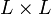$L \times L$ sites), using periodic boundary conditions. In such simulations one can generate different system realizations for given values of$x$, and compute the fraction,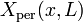$X_{\rm per}(x,L)$, of realizations with percolating clusters. For low values of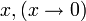$x, ( x \rightarrow 0 )$ one will have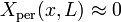$X_{\rm per}(x,L) \approx 0$, whereas when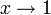$x \rightarrow 1$, then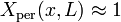$X_{\rm per}(x,L) \approx 1$. Considering the behavior of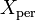$X_{\rm per}$ as a function of$x$, for different values of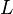$L$ the transition between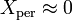$X_{\rm per} \approx 0$ and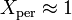$X_{\rm per} \approx 1$ occurs more abruptly as$L$ increases. In addition, it is possible to compute the value of the occupancy probability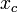$x_{c}$ at which the transition would take place for an infinite system (that is to say, in the thermodynamic limit).

### Finite-size scaling

Considering the functions$X_{\rm per}(x,L)$ the percolation theory predicts for large system sizes:

•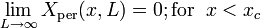$\lim_{L \rightarrow \infty} X_{\rm per}(x,L) = 0 ; {\rm for} \; \; x < x_c$
•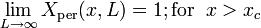$\lim_{L \rightarrow \infty} X_{\rm per}(x,L) = 1 ; {\rm for} \; \; x > x_c$

In addition, at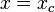$x = x_c$, it is expected that the fraction of percolating realizations do not depend on the system size:

•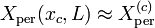$X_{\rm per}(x_c,L) \approx X_{\rm per}^{(c)}$ ; for large values of$L$.

## Computation of the percolation threshold

A couple of simple procedures to estimate the percolation threshold ($x_c$ in the example introduced above) are described here. These procedures are similar to those used in the analysis of critical thermodynamic transitions. More sophisticated methods can be found in the literature (See Refs.    for details).

### Crossing of the$X_{\rm per}(x,L)$ for different system sizes

In practice, one has to compute the fraction of percolating realizations for different values of the control parameter$\left. x \right.$ and different system sizes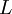$\left. L \right.$. The critical value$x_c$ is then estimated by plotting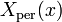$\left. X_{\rm per}(x) \right.$ as a function of$\left. x \right.$ for several values of$L$. The crossing of the curves with different values of$L$ provide estimates of both$x_c$ and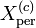$X_{\rm per}^{(c)}$.

### Computation of pseudo-critical parameters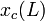$x_c(L)$ and extrapolation

Given the results of$X_{\rm per}(x,L)$ for a given system size$L$, a pseudo-critical size dependent variable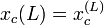$x_c(L)=x_c^{(L)}$ is computed by matching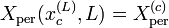$X_{\rm per}(x_c^{(L)},L) = X_{\rm per}^{(c)}$.

If the universal value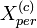$X_{per}^{(c)}$ value is unknown for the type of transition considered, an alternative definition for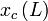$x_c\left(L \right)$ can be taken, for instance: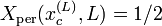$X_{\rm per}(x_c^{(L)},L) = 1/2$.

The percolation theory predicts that the pseudo-critical values$x_c(L)$ will scale as: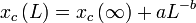$x_c \left( L \right) = x_c \left( \infty \right) + a L^{- b}$

where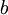$b$ is a critical exponent (See Refs.   for details). Therefore, by fitting the results of$x_c(L)$ it is possible to estimate the percolation transition location:$x_c = x_c ( \infty )$.

## Percolation threshold and critical thermodynamic transitions

In some systems, with an appropriate definition of bonding criteria, the percolation transition occurs at the same value of the control parameter (density, temperature, chemical potential) as the thermodynamic transition    . In these case cluster algorithms become very efficient, and moreover, the percolation analysis can be useful to develop algorithms to locate the transition (see the cluster algorithms page for more details).